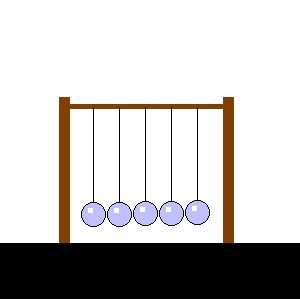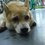# Law of conservation of MOMENTUMMeaning :

Total momentum in an isolated system does not change. the law of physics that states that the linear momentum does not change unless an external force acts upon it.

The law of conservation of momentum explains that momentum is neither lost or gained. "when two bodies collide with one another, the total energy remains constant. That means that the total amount of momentum doesn't change.

Examples :

In a collision or Newton's Cradle. (5 balls hanging on a string, when you move one to hit the other balls, there is a reaction ) . Recoil

Momentum is the concept that links the action of a force and the change of speed it produces. It accounts for the fact that a force will produce a large acceleration in a small mass but a small acceleration in a large mass. If this law was not universal, then the outcomes of identical interactions would vary,contrary to the basic tenets of physics.

Formula :

Total Momentum After Collision = Total Momentum Before Collision

             : m1u1 + m2u2 = m1v1 + m2v2               ----( 1)


Elastic and Inelastic Collisions: ΔK.E. = (Total K.E.)after collision - (Total K.E.)before collision

            : 1/2  (m1u1)^2 + 1/2 (m2u2) ^2 = 1/2(m1v1)^2 + 1/2 (m2v2) ^2    ----( 2)


Combined equation 1 & 2 to form a new formula .

TRY TO COMBINE THEM TO FORM A NEW AND USEFUL FORMULA :)Note by Nicole Ling
6 years, 6 months ago

This discussion board is a place to discuss our Daily Challenges and the math and science related to those challenges. Explanations are more than just a solution — they should explain the steps and thinking strategies that you used to obtain the solution. Comments should further the discussion of math and science.

When posting on Brilliant:

• Use the emojis to react to an explanation, whether you're congratulating a job well done , or just really confused .
• Ask specific questions about the challenge or the steps in somebody's explanation. Well-posed questions can add a lot to the discussion, but posting "I don't understand!" doesn't help anyone.
• Try to contribute something new to the discussion, whether it is an extension, generalization or other idea related to the challenge.

MarkdownAppears as
*italics* or _italics_ italics
**bold** or __bold__ bold
- bulleted- list
• bulleted
• list
1. numbered2. list
1. numbered
2. list
Note: you must add a full line of space before and after lists for them to show up correctly
paragraph 1paragraph 2

paragraph 1

paragraph 2

[example link](https://brilliant.org)example link
> This is a quote
This is a quote
    # I indented these lines
# 4 spaces, and now they show
# up as a code block.

print "hello world"
# I indented these lines
# 4 spaces, and now they show
# up as a code block.

print "hello world"
MathAppears as
Remember to wrap math in $$ ... $$ or $ ... $ to ensure proper formatting.
2 \times 3 $2 \times 3$
2^{34} $2^{34}$
a_{i-1} $a_{i-1}$
\frac{2}{3} $\frac{2}{3}$
\sqrt{2} $\sqrt{2}$
\sum_{i=1}^3 $\sum_{i=1}^3$
\sin \theta $\sin \theta$
\boxed{123} $\boxed{123}$

Sort by:

Oh nice!! The conservation of momentum is really useful sometimes. It seems like common sense, but yeah!! Well explained. (y) I also like the Newton Cradle animation. ^^

- 6 years, 5 months ago

v1-u2=u1-v2

- 6 years, 6 months ago

You can wrote like this too . It's up to you :)

- 6 years, 6 months ago

[(m2/m1) (u2 - v1)][(u2 + v2) - ((m2/m1) (u2 - v2)) - 2 u1]=0 i don't know if this formula is useful or not but this what i've got by isolating v1 it depends on what data you have been given nice topic to discuss...

- 6 years, 6 months ago

The formula that you have written is useful too :) It's depend on how you use it and you can think of what types of question is suitable to use the formula that you created... So you can solve the problem easier and faster :)

- 6 years, 6 months ago

v1 - v2 = u2- u1

- 6 years, 6 months ago

It's right too :) It's depends on how you use it .

- 6 years, 6 months ago

Hi Nicole. You must be in Form 5 (SPM)?

- 6 years, 6 months ago

Ya , How did u know it :) ?

- 6 years, 6 months ago

I took my SPM in 1984. I am not a 20 year old by a long shot.

- 6 years, 6 months ago

That's what is required from a 17 year old from Malaysia. (plus I know everything) Haha

- 6 years, 6 months ago

lol haha

- 6 years, 6 months ago